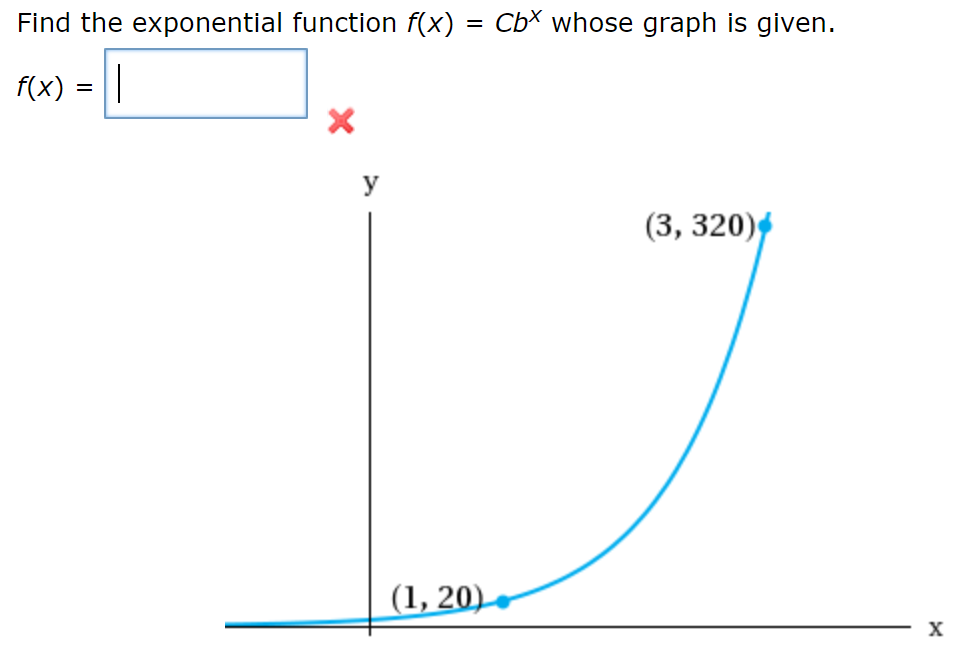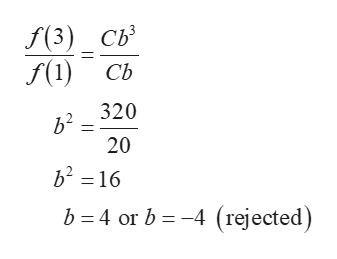Find the exponential function f(x) = CbX whose graph is given.f(x)_y(3, 320)(1, 20)X

Questionhelp_outlineImage TranscriptioncloseFind the exponential function f(x) = CbX whose graph is given. f(x) _ y (3, 320) (1, 20) X fullscreen
Step 1

Consider the given exponential function as f(x)=Cbx.

The curve of the function passes through the points (1, 20) and (3, 320).

Hence, the value of function at the points 1 and 3 are 20 and 320, respect...help_outlineImage TranscriptioncloseF(3) св S() сь 2 320 20 b2 16 b 4 or b-4 (rejected fullscreen

Want to see the full answer?

See Solution

Want to see this answer and more?

Our solutions are written by experts, many with advanced degrees, and available 24/7

See Solution
Tagged in

Other